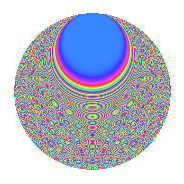# Properties

 Label 430.2.xLevel 430 Weight 2 Character orbit x Rep. character $$\chi_{430}(3,\cdot)$$ Character field $$\Q(\zeta_{84})$$ Dimension 528 Newform subspaces 1 Sturm bound 132 Trace bound 0

# Related objects

## Defining parameters

 Level: $$N$$ = $$430 = 2 \cdot 5 \cdot 43$$ Weight: $$k$$ = $$2$$ Character orbit: $$[\chi]$$ = 430.x (of order $$84$$ and degree $$24$$) Character conductor: $$\operatorname{cond}(\chi)$$ = $$215$$ Character field: $$\Q(\zeta_{84})$$ Newform subspaces: $$1$$ Sturm bound: $$132$$ Trace bound: $$0$$

## Dimensions

The following table gives the dimensions of various subspaces of $$M_{2}(430, [\chi])$$.

Total New Old
Modular forms 1680 528 1152
Cusp forms 1488 528 960
Eisenstein series 192 0 192

## Trace form

 $$528q - 4q^{6} - 12q^{7} + O(q^{10})$$ $$528q - 4q^{6} - 12q^{7} + 40q^{13} + 88q^{16} - 4q^{17} + 16q^{21} - 12q^{23} + 8q^{25} - 12q^{28} + 36q^{30} - 72q^{31} - 124q^{33} + 40q^{35} - 268q^{36} - 44q^{38} + 56q^{41} - 168q^{43} - 24q^{46} - 72q^{47} - 24q^{50} + 280q^{51} + 16q^{52} - 132q^{53} - 24q^{55} + 8q^{56} - 20q^{57} - 8q^{60} - 72q^{61} + 48q^{62} - 84q^{65} - 72q^{66} - 40q^{67} - 32q^{68} - 24q^{71} + 192q^{73} + 48q^{76} - 148q^{77} - 40q^{78} + 56q^{82} - 28q^{83} - 20q^{86} - 216q^{87} + 8q^{90} - 48q^{91} + 12q^{92} + 108q^{93} - 4q^{95} + 4q^{96} - 120q^{97} + 96q^{98} + O(q^{100})$$

## Decomposition of $$S_{2}^{\mathrm{new}}(430, [\chi])$$ into newform subspaces

Label Dim. $$A$$ Field CM Traces $q$-expansion
$$a_2$$ $$a_3$$ $$a_5$$ $$a_7$$
430.2.x.a $$528$$ $$3.434$$ None $$0$$ $$0$$ $$0$$ $$-12$$

## Decomposition of $$S_{2}^{\mathrm{old}}(430, [\chi])$$ into lower level spaces

$$S_{2}^{\mathrm{old}}(430, [\chi]) \cong$$ $$S_{2}^{\mathrm{new}}(215, [\chi])$$$$^{\oplus 2}$$

## Hecke Characteristic Polynomials

There are no characteristic polynomials of Hecke operators in the database